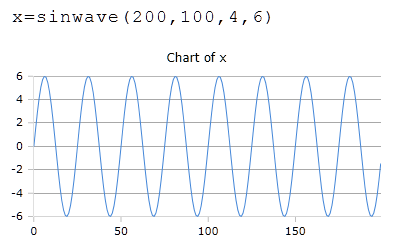# SinWave Function

Generates a sinusoidal curve

## Description

The function SinWavecreates a series of numbers that represents a sinusoid, starting with the positive half-wave.

### Syntax

SinWave (Length, SamplingRate, Frequency, Amplitude)

SinWave (Length, SamplingRate, Frequency, Amplitude, Mean)

SinWave (Length, SamplingRate, Frequency, Amplitude, Mean, Phase)

SinWave (Length, SamplingRate, Frequency, Amplitude, Mean, Phase, Delay)

### Parameters

Length

The number of samples to generate

Samples

Samples per time unit. This means that the value corresponds to the total number of samples for representing all periods specified under Frequency. Must be greater than twice the frequency.

Frequency

Frequency in periods per time unit (Hz)

Amplitude

The maximum peak

### Optional Parameters

Mean

DC component part of the signal.

Phase

Offset of the phase.

Delay

Delay, relative to the phase.

### Example